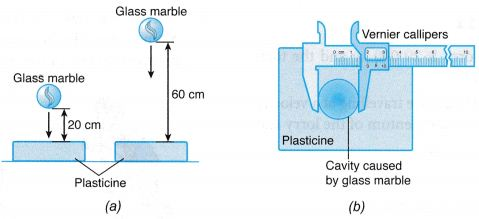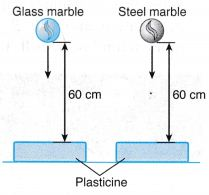## What Is Momentum1. Every moving object has momentum. However, the momentum of a timber lorry is very much bigger than that of a cyclist, despite them moving at the same speed.
2. The product of mass and velocity is called ‘momentum‘.
$$\text{i}\text{.e}\text{. }p=m\overset{\to }{\mathop{v}}$$
3. Unit: SI unit of momentum is kg-m/s.
4. It is a vector quantity.

### Activity 1

Aim: To study the effect of stopping two objects
A. of the same mass moving at different speeds
B. of different masses moving at the same speed
Materials: Two identical glass marbles, soft plasticine, a steel marble of the same size as the glass marbles but with a bigger mass
Apparatus: Meter ruler, vernier callipers
Method:
A. Same Mass Moving at Different Speeds1. Two identical pieces of soft plasticine are placed on a table.
2. A glass marble is dropped on the plasticine from a height of 20 cm. Another identical glass marble is dropped on the other piece of plasticine from a height of 60 cm as shown in Figure (a).
3. The diameter of the cavity on the surface of each plasticine caused by the falling marbles is measured using a pair of vernier callipers and compared.

B. Different Masses Moving at the Same Speed1. The method in section A is repeated by releasing a glass marble and a steel marble of the same size, both from a height of 60 cm.

Results:
A. Same Mass Moving at Different Speeds
The diameter of the cavity on the surface of the plasticine caused by the glass marble that is dropped from a height of 60 cm is larger than that caused by the glass marble that is dropped from a height of 20 cm.
B. Different Masses Moving at the Same Speed
The diameter of the cavity on the surface of the plasticine caused by the steel marble is larger than that caused by the glass marble.
Discussion:
A larger cavity on the surface of the plasticine caused by a falling marble means a bigger effect on the plasticine in stopping the marble. The size of the cavity is a measure of the magnitude of the momentum of the moving object.
A. Same Mass Moving at Different Speeds

1. The glass marble that is dropped from a higher height will reach the surface of the plasticine with a higher speed.
2. Both marbles are identical and hence, they have the same mass. Therefore, for two objects of equal mass, stopping the one with a higher speed requires greater effort than the one with a lower speed.

B. Different Masses Moving at the Same Speed

1. Both the glass marble and the steel marble are dropped from the same height. Therefore, both of them will reach the surface of the plasticine with the same velocity.
2. The steel marble has a greater mass than the glass marble. Therefore, for two objects of different masses but moving at the same speed, the effect of stopping the one with a bigger mass is greater than the one with a smaller mass.

Conclusion:
The linear momentum of an object depends on its mass and speed. For an object with constant mass, the higher its speed, the higher is its momentum. For an object with constant speed, the larger its mass, the higher is its momentum.

## Momentum Example Problems With Solutions

Example 1. Calculate the force required to produce an acceleration of 5 m/s2 in a body of mass 2.4 kg.
Solution:    We know that force = mass × acceleration
= 2.4 kg × 5 m/s2
= 12.0N

Example 2. A body of mass 2.5 kg is moving with a velocity of 20 m/s. Calculate its momentum.
Solution:    Momentum, p = mass × velocity
Here, mass m = 2.5 kg
Velocity, v = 20 m/s
∴ Momentum, p = mv = 2.5 × 20 kg-m/s
= 50 kg-m/s

Example 3. The total mass of a lorry is 20000 kg and the total mass of a car is 2000 kg.
If both the lorry and the car are travelling at a velocity of 25 m s-1, calculate the momentum of the lorry and the car respectively.
Solution:
Momentum of the lorry = 20000 × 25
= 5 × 105 kg m s-1
Momentum of the car = 2000 × 25
= 5 × 10 kg m s-1